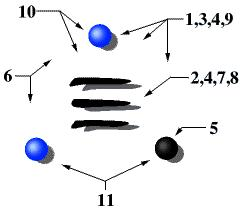Text Size: ππππ

CECM Home > About Us > CECM's Logo

# CECM's Logo

## What does it mean anyways?1. The three spheres suggest the informal mathematical therefore symbol, referring to the CECM's interest in making experimental mathematics an effective approach.
2. The three lines suggest the mathematical symbol equivalent to, again implying that experimental mathematics will yield results as concrete and far-reaching as conventional mathematics.
3. The three spheres represent the three active groups at the CECM; the Number Theory Group, the Computational Algebra Group, and the Discrete Mathematics Group.
4. Both sets of three suggest the three founding members of the CECM, Jon Borwein, Peter Borwein and Loki Jörgenson.
5. The single black sphere represents 1.
6. The two blue spheres represent 2.
7. The three lines represent 3. They are also the Sanskrit symbol for 3; Sanskrit is the language of the first mathematics.
8. The three lines are also the Chinese trigram for strength.
9. The three spheres suggest the three aspects of science as it is currently employed: Theory (thought), experiment (physical) and simulation (computation). Or more appropriately to mathematics which is inherently abstract; theory, application and experiment. The black sphere is the focus of the CECM, experiment using computer.
10. The perfect equilateral triangle contructed from the three perfect spheres suggests precision and formal rigour. The three apparently hand-painted lines suggest human endeavour, creativity and personal effort.
11. The colours of the CECM are blue (for the sky) and black (for readability).